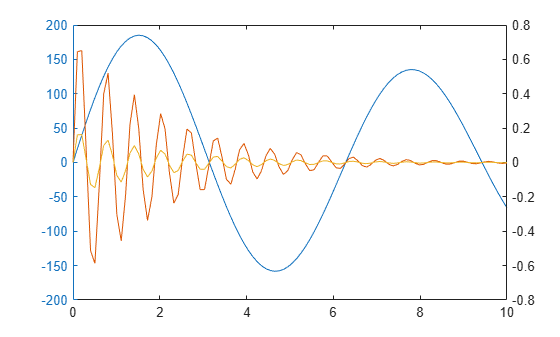# plotyy

(Not recommended) Create graph with two y-axes

`plotyy` is not recommended. Use `yyaxis` instead. For more information, see Compatibility Considerations.

## Syntax

```plotyy(X1,Y1,X2,Y2) plotyy(X1,Y1,X2,Y2,function) plotyy(X1,Y1,X2,Y2,'function1','function2') plotyy(AX1,___) [AX,H1,H2] = plotyy(___) ```

## Description

`plotyy(X1,Y1,X2,Y2)` plots `Y1` versus `X1` with y-axis labeling on the left and plots `Y2` versus `X2` with y-axis labeling on the right.

`plotyy(X1,Y1,X2,Y2,function)` uses the specified plotting function to produce the graph.

`function` can be either a function handle or a character vector specifying `plot`, `semilogx`, `semilogy`, `loglog`, `stem`, or any MATLAB® function that accepts the syntax

`h = function(x,y)`

For example,

```plotyy(x1,y1,x2,y2,@loglog) % function handle plotyy(x1,y1,x2,y2,'loglog') % character vector```

Function handles enable you to access user-defined local functions and can provide other advantages. For more information on using function handles, see Create Function Handle.

`plotyy(X1,Y1,X2,Y2,'function1','function2')` uses `function1(X1,Y1)` to plot the data for the left axis and `function2(X2,Y2)` to plot the data for the right axis.

`plotyy(AX1,___)` plots the data using the axes specified by `AX1` for the first set of data, instead of using the current axes. Specify `AX1` as a single axes object or a vector of the two axes objects returned by a previous call to `plotyy`. If you specify a vector, then `plotyy` uses the first axes object in the vector. Use this option with any of the input argument combinations in the previous syntaxes.

`[AX,H1,H2] = plotyy(___)` returns the handles of the two axes created in `AX` and the handles of the graphics objects from each plot in `H1` and `H2`. `AX(1)` is the left axes and `AX(2)` is the right axes.

## Examples

collapse all

Plot two data sets on one graph using two y-axes.

```x = 0:0.01:20; y1 = 200*exp(-0.05*x).*sin(x); y2 = 0.8*exp(-0.5*x).*sin(10*x); figure % new figure plotyy(x,y1,x,y2)```Plot two data sets using a graph with two y-axes. Add a title and axis labels.

```x = 0:0.01:20; y1 = 200*exp(-0.05*x).*sin(x); y2 = 0.8*exp(-0.5*x).*sin(10*x); figure % new figure [hAx,hLine1,hLine2] = plotyy(x,y1,x,y2); title('Multiple Decay Rates') xlabel('Time (\musec)') ylabel(hAx(1),'Slow Decay') % left y-axis ylabel(hAx(2),'Fast Decay') % right y-axis```Plot two data sets using a graph with two y-axes. Change the line styles. Starting in R2014b, you can use dot notation to set properties. If you are using an earlier release, use the `set` function instead.

```x = 0:0.01:20; y1 = 200*exp(-0.05*x).*sin(x); y2 = 0.8*exp(-0.5*x).*sin(10*x); [hAx,hLine1,hLine2] = plotyy(x,y1,x,y2); hLine1.LineStyle = '--'; hLine2.LineStyle = ':';```Plot two data sets using a graph with two y-axes. Use a line plot for the data associated with the left y-axes. Use a stem plot for the data associated with the right y-axes.

```x = 0:0.1:10; y1 = 200*exp(-0.05*x).*sin(x); y2 = 0.8*exp(-0.5*x).*sin(10*x); figure plotyy(x,y1,x,y2,'plot','stem')```Plot three data sets using a graph with two y-axes. Plot one set of data associated with the left y-axis. Plot two sets of data associated with the right y-axis by using two-column matrices.

```x = linspace(0,10); y1 = 200*exp(-0.05*x).*sin(x); y2 = 0.8*exp(-0.5*x).*sin(10*x); y3 = 0.2*exp(-0.5*x).*sin(10*x); figure [hAx,hLine1,hLine2] = plotyy(x,y1,[x',x'],[y2',y3']);```## Version History

Introduced before R2006a

expand all

Not recommended starting in R2016a